[ezcol_1third]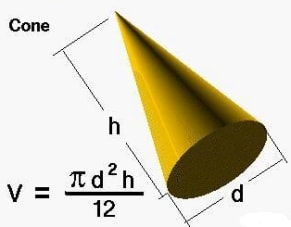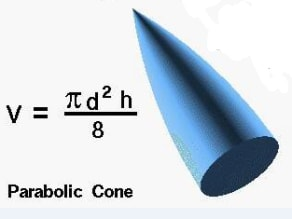[/ezcol_1third]

[ezcol_1third]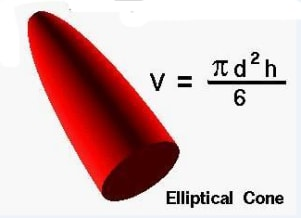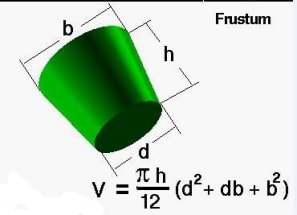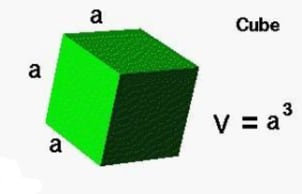[/ezcol_1third]

[ezcol_1third_end]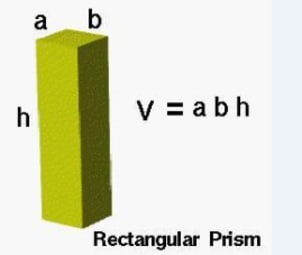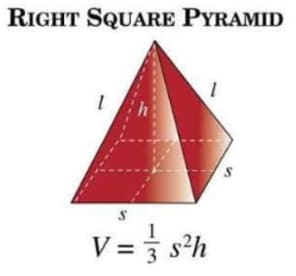[/ezcol_1third_end]

Volume is the three-dimensional space a substance occupies. Volumes of many simple shapes can be calculated by using simple formulas. More complicated shapes sometimes can be cut into simple shapes and add the volumes up for the total. Some other complicated shapes can be calculated by integral calculus if a formula exists for the shape’s boundary. The volume of even more complicated shapes can be estimated with mathematical methods, such as finite element method. If the density is uniform, the volume of a substance can be calculated from the weight.